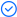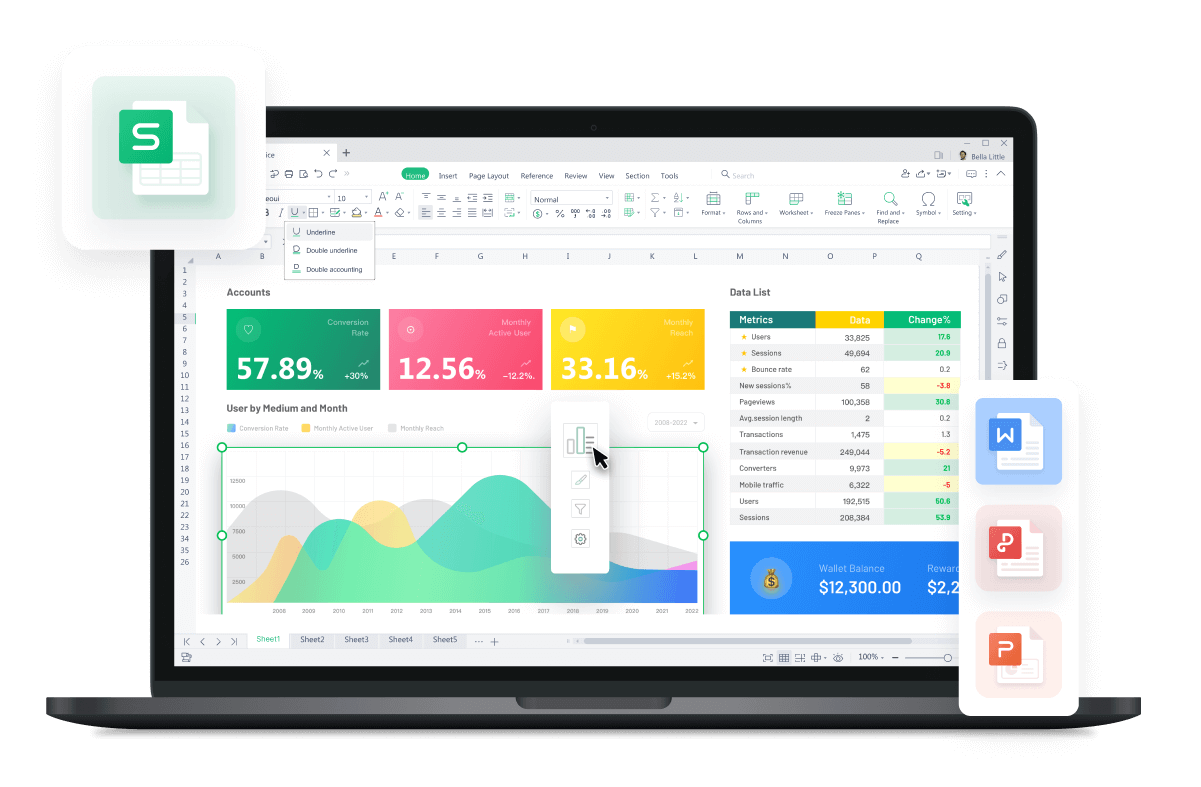WPS Office

Free All-in-One Office Suite with PDF EditorRead, edit, and convert PDFs with the powerful PDF toolkit.Microsoft-like interface, easy to use.

Windows • MacOS • Linux • iOS • Android# Use subtotal feature to do statistic

Uploaded time: December 1, 2021 Difficulty Beginner

# Use subtotal feature to do statisticUse subtotal feature to do statistic

The Subtotal feature of the WPS Spreadsheet is a commonly used feature when processing data. It can automatically statistic the data in the worksheet by fields. So how can we use this feature?

Take this table as an example.

Before using the subtotal feature, we need to edit the table a little bit. Because when using the subtotal function, we must first sort the fields to be classified, otherwise the result of subtotal may be inconsistent with the expected effect.

1. Select any cell in the Type of Expense column.

2. Click the Data tab.

3. Then click the Sort drop-down button, and select Ascending.

4. After sorting, the data of the same field is gathered together. Now it will be clearer for us when we use the subtotal feature.

Now I have a reimbursement list with a large amount of data. Let's suppose we want to sort data according to the Type of Expense field and summarize the data of Expense details. How can we do that?

1. Click the Subtotal button in the Data tab.

2. Now in the Subtotal dialog box, select Type of Expense in the At each change in area.

3. Select Sum in Use function.

4. Check the Expense details option in Add subtotal to.

At the bottom of the dialog box, we can see three options selected by default, namely Replace current subtotals, Summary below data, and Filter subtotal. There are two more options in the dialog box: Copy subtotal to a new sheet and Page break between groups. Now I'm going to explain the functions of these options one by one.

· 1. Replace current subtotals

Now the form has already been classified and summarized by the Account Number field. We may want to change it to the Type of Expense field. Check Replace current subtotals, then click OK to replace the original subtotals table.

· 2. Summary below data

We can check Summary below data according to personal preference so that the summary results will be displayed below each set of data.

If we uncheck Summary below data in the Subtotal dialog box, and click OK, then the summary result will be displayed above each set of data.

After that, we may find that the resulting subtotal table was not what we wanted. We can click the Subtotal button and click Remove All to restore to the table before we used the subtotal feature.

· 3. Filter subtotal

If we check Filter subtotal in the Subtotal dialog box, the table will retain the original worksheet and create a new subtotal worksheet.

· 4. Copy subtotal to a new sheet

Open the Subtotal dialog box, check Copy subtotal to a new sheet, then the table will create a new worksheet according to the classification of the subtotal.

Here is a serial number in the upper left corner of the worksheet. Click the serial number in the upper left corner of the worksheet, and we can expand the corresponding classification data. The table will create a new worksheet according to the expanded classification of the subtotal.

· 5. Page break between groups

What if we want to group and print the contents of the Type of Expense field? Open the Subtotal dialog box, check Page break between groups, and then click OK. In the new worksheet, we select all the cells and adjust the table to the appropriate column width. Click Print Preview in the quick access toolbar, and then we can see that the Spreadsheet has divided the contents of the Type of Expense field into pages.

To make it easier for us to view the printed subtotal data, we can add a header title to each page of Print Preview. Click the Page Layout tab, then click the Print Titles button. Click Sheet in the popup dialog box, then select the first row in the Print titles edit box, and then click OK. Now we go back to Print Preview. Then we can see that the content of each page has a header title.

Above is the introduction on the subtotal feature. It is very powerful and practical. Did you get it?

This skill could be also used in Microsoft Office Excel and Openoffice.